﻿ 某高层建筑物纠偏加固设计与施工技术研究 Research on the Design and Construction Technology of Rectification and Reinforcement of a High-Rise Building

Hans Journal of Civil Engineering
Vol. 08  No. 01 ( 2019 ), Article ID: 28596 , 6 pages
10.12677/HJCE.2019.81017

Research on the Design and Construction Technology of Rectification and Reinforcement of a High-Rise Building

Weifeng Xu1, Shuai Li2, Yaping Ren2

1Nanjing Golden Eagle International Group Co., Ltd., Nanjing Jiangsu

2College of Civil Engineering, Nanjing University of Technology, Nanjing Jiangsu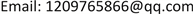Received: Jan. 1st, 2019; accepted: Jan. 16th, 2019; published: Jan. 23rd, 2019ABSTRACT

This project is a high-rise rectification and reinforcement project, shear wall structure, using the raft foundation. Tilting the whole of the south occurred after the completion of the building. In this paper, through the analysis of the cause of the tilt, combined with the actual situation of the building, the radiation well water correction method is used, combined with the anchor static pressure pile to carry out the foundation reinforcement. The design, implementation and correctiveness monitoring of the corrective deviation scheme have been introduced in detail, which provides guarantee for the successful correction of the later period, and also provides reference for other similar correction projects.

Keywords:Water Jetting Rectification, Anchor Jacked Pile, Rectification Monitoring

1南京金鹰国际集团有限公司，江苏 南京

2南京工业大学土木工程学院，江苏 南京Copyright © 2019 by authors and Hans Publishers Inc.1. 引言

2. 工程概况

3. 建筑倾斜原因分析

3.1. 工程地质情况Table 1. Site soil layer and its physical parameters

3.2. 倾斜原因分析

3.2.1. 地基不均匀沉降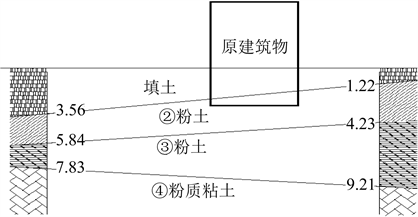Figure 1. Typical geological profile

3.2.2. 施工因素

4. 纠倾加固方案

4.1. 方案概述

4.2. 地基加固设计

${Q}_{uk}={\mu }_{p}{\sum }^{\text{​}}\left({q}_{sia}×{l}_{i}\right)+{q}_{pa}×{A}_{p}$ (1)

${Q}_{uk}=1.2×\left(35×2.5+40×3.51+45×1.47+50×1.35+95×1.0\right)+4000×0.09=949.86\text{kN}$

4.3. 辐射井射水纠偏设计

$\Delta s=\left({i}_{实}-{i}_{控}\right)×L=\left(7%-3%\right)×16762=67.05\text{mm}$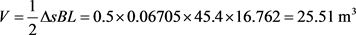$V=n{\sum }^{\text{​}}\partial \frac{31}{3}\text{π}{r}_{i}^{2}{l}_{i}=27×0.8×\frac{31}{3}×\text{π}×{0.075}^{2}×8=31.54{\text{m}}^{3}$ ，满足要求。

4.4. 纠偏防复倾措施

5. 施工工艺及监测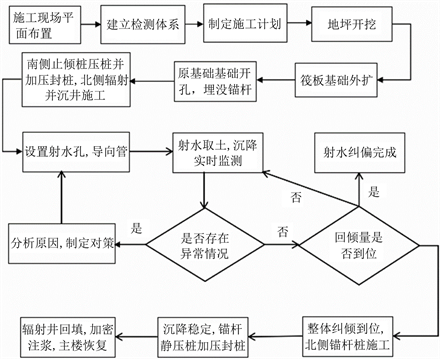Figure 2. Process flow chart of rectifying deviation construction

6. 纠偏监测

6.1. 监测点布置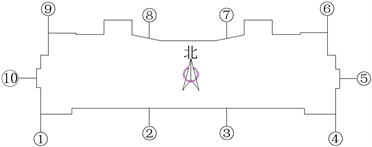Figure 3. Settlement observation point layout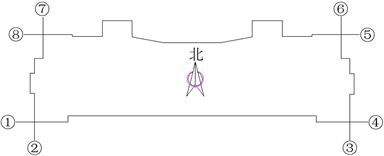Figure 4. Tilted observation point layout

6.2. 沉降监测结果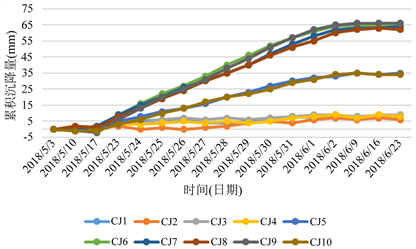Figure 5. Settlement change curve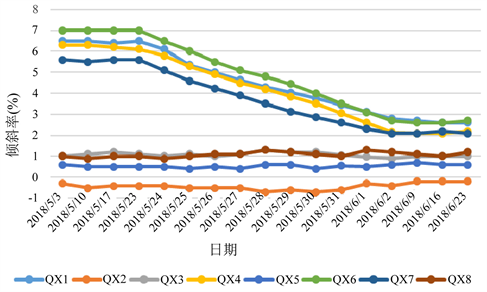Figure 6. Skew change curve

7. 结论

Research on the Design and Construction Technology of Rectification and Reinforcement of a High-Rise Building[J]. 土木工程, 2019, 08(01): 131-136. https://doi.org/10.12677/HJCE.2019.81017

1. 1. 何文华. 压桩掏土纠偏法在某倾斜房屋纠偏加固中的应用[J]. 广东土木与建筑, 2012(4): 62-64.

2. 2. 王玉珏, 李延和, 任亚平. 锚杆静压桩承载力计算方法及基桩检测技术研究[D]: [硕士学位论文]. 南京: 南京工业大学, 2009.

3. 3. 郑志远, 李延和. 锚杆静压桩承载力计算方法及基桩检测技术研究[D]: [硕士学位论文]. 南京: 南京工业大学, 2013.

4. 4. 李启民. 建筑物纠倾工程设计与施工[M]. 北京: 中国建筑工业出版社, 2012.